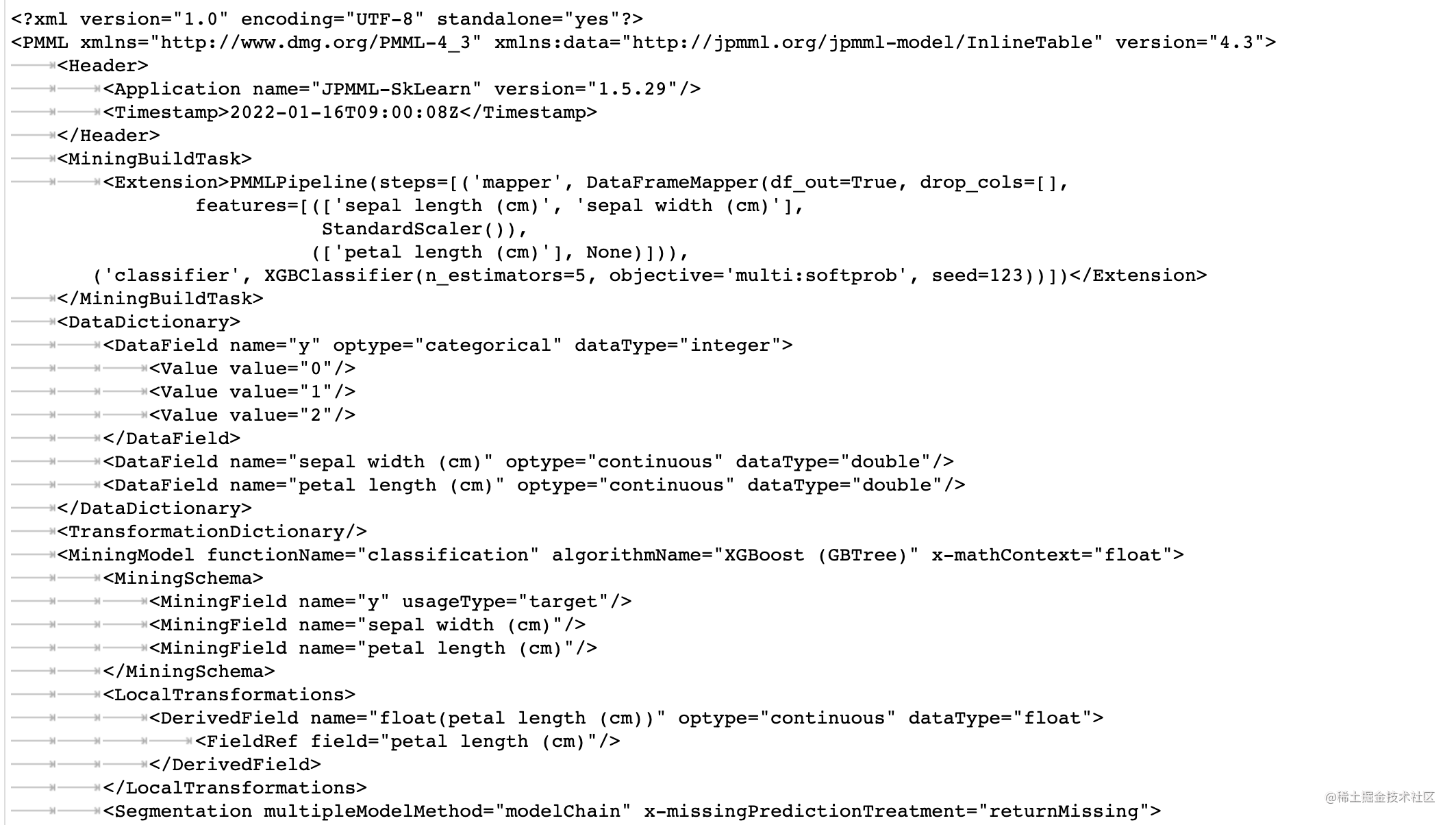# PMML部署演算法模型

#### 1. PMML是什麼? 🤔

PMML是一套基於XML標準，與平臺和環境無關的模型表示語言。它主要是通過了XML schema定義和儲存了一個演算法模型的核心元素：

• 資料字典：描述輸入資料
• 資料轉換：定義了原始資料資料預處理的方式，比如標準化、缺失值處理、啞變數的生成等
• 模型定義：模型的型別及引數，比如樹模型的分裂節點等
• 模型輸出：模型的輸出結果

#### 2. Python怎麼實現PMML檔案打包? 💻

`Iris`鳶尾花資料集，包含4個特徵變數（sepal length, sepal width, petal length和petal width）以及1個類別標籤（0-setosa, 1-versicolor, 2-virginica），我們的模型目標是：基於特徵變數，將3類鳶尾花區分開。

```python

# import packages

import pandas as pd import numpy as np from sklearn import datasets from sklearn.model_selection import train_test_split from sklearn_pandas import DataFrameMapper from sklearn.preprocessing import StandardScaler from sklearn2pmml import PMMLPipeline from sklearn2pmml import sklearn2pmml from xgboost import XGBClassifier ```

##### Step 1: 使用python訓練一個模型

```python

iris = datasets.load_iris() X = pd.DataFrame(iris.data, columns=iris.feature_names) y = iris.target

# split train and test dataset

X_train, X_test, y_train, y_test = train_test_split(X, y, test_size=0.2, random_state=123)

# define datamapper

mapper = DataFrameMapper([ (["sepal length (cm)", "sepal width (cm)"], StandardScaler()), (["petal length (cm)"], None) ], df_out=True)

# define a model

xgb = XGBClassifier(n_estimators=5, seed=123)

# make a pipeline

pip_model = PMMLPipeline([ ('mapper', mapper), ("classifier", xgb)] )

# train a model & predict on test data

pip_model.fit(X_train, y_train) pred_prob_pip = pip_model.predict_proba(X_test) ```

```python

# save model

sklearn2pmml(pip_model, "iris_model.pmml", with_repr = True) ```##### Step 3: python中呼叫PMML校驗結果

```python from pypmml import Model model = Model.fromFile('iris_model.pmml') pred_prob_reloaded_model = model.predict(X_test)```

#### 3. 可能會遇到什麼坑？😂

• 坑1: 可以訓練模型但是卻不能生成pmml檔案

可能是由於環境原因導致，sklearn/sklearn2pmml/sklearn_pandas需要適配，而且當sklearn2pmml版本過高而打jar版本過低，則會導致檔案無法讀取的問題，本文使用版本資訊如下：

• sklearn2pmml = 0.53.0
• sklearn = 0.23.1
• sklearn_pandas = 2.2.0
• 坑2: Java呼叫PMML和原Pipeline模型預測不一致（正常情況下，小數點後5位應該保持一致

導致這個問題的原因可能是兩個： （1）資料型別不一致；（2）缺失值的處理。

• 資料型別不一致，可以嘗試以下2個解決方案：

• 因為生成pmml檔案時，所有的變數預設是double型別，而有些分類變數是string型別，所以可以考慮對分類變數進行labelencoder處理成數值型
• 將pmml檔案中double改成string
• 缺失值的處理：當資料中存在缺失值，且不是為nan，而是以-9999代替時，PMML檔案需要在datamapper和模型中同時定義`-9999`為缺失值， 否則就會出現預測不一致現象。此時應該

```python

# define datamapper

mapper = DataFrameMapper([ (["sepal length (cm)", "sepal width (cm)"], StandardScaler()), (["petal length (cm)"], ContinuousDomain(missing_values=-9999.0, with_data = False)) ], df_out=True)

# define a model

xgb = XGBClassifier(n_estimators=5, seed=123, missing=-9999.0) ```

注：使用`ContinuousDomain`後會根據訓練資料找到每個特徵的資料範圍，而當測試資料數顯超出此範圍的數值後會報錯，因此需要設定`with_data=False`來去除這一限制。

• 坑3: 原Pipeline模型和python讀取PMML檔案模型預測結果不一致

這種情況很大程度上也和缺失值有關。如果在正確處理坑2的後，還出現此類問題，可能是由於python讀取PMML檔案模型預測的時候，對於缺失特徵也傳入並賦值`-9999`。正確做法是，使用pypmml呼叫PMML檔案，應該對缺失特徵不傳入，或者賦值為nan，這樣pypmml呼叫PMML檔案和原Pipeline模型預測結果就能保持一致。

#### 敬請期待

• Python構造Pipeline模型
• 使用Scala呼叫python訓練的PMML

「其他文章」